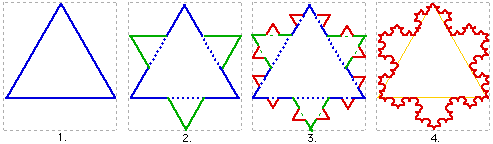### Marginal Analysis of the Fractal

Marginalism and Marginal Analysis : derived from the fractal.
Here I preform basic marginal analysis on the Koch Snowflake
Update May 2017
This is by far my best idea; I have written it up in a working paper at my academia.edu and vixra, and named it:  Quantum Mechanics, Information and Knowledge, all Aspects of Fractal Geometry and Revealed in an Understanding of Marginal Economics.
I shall post the Abstract, followed by the original post. I hope to have some collaborate and review my work in time.

Abstract
Fractal geometry is found universally and is said to be one of the best descriptions of our reality – from clouds and trees, to market price behaviour. As a fractal structure emerges – the repeating of a simple rule – it appears to share direct properties familiar to classical economics, including production, consumption, and equilibrium. This paper was an investigation into whether the mathematical principles behind ‘the market’ – known as marginalism – is an aspect or manifestation of a fractal geometry or attractor. Total and marginal areas (assumed to stand for utility) and the cost of production were graphed as the fractal grew and compared to a classical interpretation of diminishing marginal utility theory, and the market supply and demand. PED and PES was also calculated and analysed with respect to (iteration) time and decay.  It was found the fractal attractor demonstrates properties and best models classical economic theory and from this it was deduced the market is a fractal attractor phenomenon where all properties are inextricably linked. The fractal, at equilibrium, appears to be a convergent – zeta function – series, able to be described by Fourier analysis, and involves Pi, i, e, 0, and 1 (of Euler’s identity) in one model. It also demonstrated growth, development, evolution and Say’s Law – production before consumption. Insights from the fractal on knowledge and knowing are also revealed, with implications on the question of what exactly is ‘science’ – and what is ‘art’? A connect between reality and quantum mechanics was identified. It was concluded marginal, classical economics is an aspect of a fractal geometry.
Keywords
Marginal, Fractal, Elasticity, Utility, Cost, Production, Price, Growth and Development, Say’s Law

Intro
For some time now, I have pondered on how the Koch Snowflake fractal (seen below) demonstrates, uncannily, key features in economic theory.
Is it that the economy - as we experience it - is a fractal? or, is the fractal an economy?
I am now confident - after doing the following basic math's - that fractal geometry is the foundation to the social science, Economics.Koch Snowflake animation:

Towards Equilibrium
From the outset, the concept of Marginalism and fractals have something in common, they are both about the next -the next unit, or the next iteration.

Once a rule, in this case new triangles,  is set in action and allowed to progress (iterate) step by step, it will go on to eventually form a snowflake.  After iteration 4, the shape will set and no longer change - at least from the viewers' static perspective.Koch Snowflake development

If one were to continue the iterations beyond the point of equilibrium (4.) the computer generating the pattern would soon crash:  the effort (cost) would multiply while the extra benefit to the viewer - in terms of the shape – would fall close to, but never, zero.
Take the time to look at this webpage http://mathforum.org/mathimages/index.php/Koch_Curve where you can experience the fill working of the Koch Curve first hand.

Method.
I analysed the growth of the Koch Snowflake using marginal analysis (a method found in any basic economic textbook) which looks at (in this case) the extra 'area' added - iteration by iteration.

Working from internet sources I constructed a table showing the area progression of the curve - iteration after iteration:

Taking s as the side length, the original triangle area is . The side length of each successive small triangle is 1/3 of those in the previous iteration; because the area of the added triangles is proportional to the square of its side length, the area of each triangle added in the nth step is 1/9th of that in the (n-1)th step. In each iteration after the first, 4 times as many triangles are added as in the previous iteration; because the first iteration adds 3 triangles, the nth iteration will add triangles. Combining these two formulae gives the iteration formula:

Area (A) of each triangle (as in column 2) = (s^2 √(3))/4

To show that the fractal Koch Snow Flake demonstrates marginal utility theory, I substituted the Economists' utility (satisfaction or benefit gained from another iteration) with the area of the curve: reasoning that after more iterations, one gets more total satisfaction or in this case Total Area (TA). One gets (pleasingly) closer to the true shape of the snowflake.
I have used no units to measure Area, as the area in the diagram corresponds to a curve I used as a reference, http://math.rice.edu/~lanius/frac/koch3.html on the internet.

Results Table

This result table will be central to my analysis of the fractal.